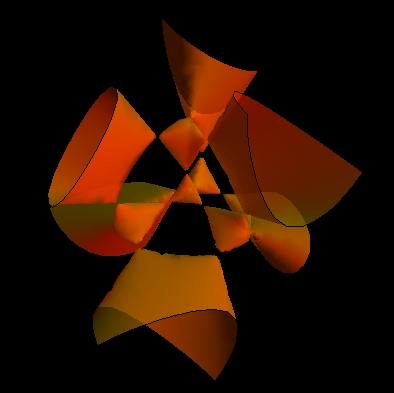M. Schilling catalog

# Kummer surface with 16 real double points

Unlike cubics, quartic surfaces have not been classified. Therefore we will be talking about some particular types of quartics.

Quartic surface (or quartic) is an algebraic surface of order four in the projective space $\mathbb{P}^3$ (real projective space $\mathbb{RP}^3$). It was proved by Kummer in 1864 that the maximum possible number of ordinary double points (also called $A_1$) for a surface of degree four is 16. Kummer surfaces are examples of quartic surfaces which have exactly 16 double points.

Kummer considered, as today called, Kummer's surfaces with tetrahedral symmetry. These surfaces are the family of 2-parameter surfaces given by

$\left[\varphi_{\mu}(x, y, z)\right]^2 - \lambda\,pqrs =0,$

where $\varphi_{\mu}=0$ is an equation of a sphere with the radius equals to $\mu$, and $pqrs = 0$ is the equation of a tetrahedron concentric with the sphere. The four sides of the tetrahedron have equations

$p(x, y, z) = 0, \ q(x, y, z) = 0, \ r(x, y, z) = 0, \ s(x, y, z) = 0.$

The parameter $\lambda$ shows how the equation of the sphere and the tetrahedron are mixed. Depending on the parameters $\mu$ and $\lambda$, the resulting surface described by the equation above may have many interesting singularities. It all depends on how the sphere $\varphi_\mu$ meets the edges of the tetrahedron $pqrs=0$. The parameter $\mu$ determines the type and locations of the singularities, and the parameter $\lambda$ determines the shape of the surface with these singularities.

In the Cartesian coordinates the equation $\varphi_\mu$ of the sphere is

$\varphi_{\mu} = x^2 + y^2 + z^2 -\mu k^2.$

We can also assume that the equations of the tetrahedron are

$p = z - k + x\sqrt{2} \quad q = z - k - x\sqrt{2}$

$r = z + k + x\sqrt{2} \quad s = z + k - x\sqrt{2}.$

Hence, in the Cartesian coordinates the equation of Kummer's surfaces with tetrahedral symmetry is

$\left(x^2 + y^2 + z^2 -\mu k^2\right)^2 = \lambda \left[(z - k)^2 - 2z^2\right]\left[(z + k)^2 - 2y^2\right].$

The constant $k$ determines the size of the particular model.

What is now known as the Kummer surfaces is the series of quartic surfaces which can be given by 2-parameter family the equation above with the parameter

$\lambda = \frac{3\mu - 1}{3 - \mu}$.

The Kummer configuration of 16 double points and 16 planes is the arrangement of points and planes in $\mathbb P^3$ such that each plane contains 6 of double points and through each double point pass 6 of the planes. Such planes are also called double planes. The intersection of the Kummer surface and each double plane is a curve of degree 2 (quadric) (see lines on the model above) in the plane, and the plane is tangent to the surface at each point of the intersection.

The model above shows the Kummer surface with all real 16 double points and planes. For it $1 < \mu < 3$. All 16 points on this model are singularities at the vertices of five tetrahedrons. The six lowest double points lie in the same plane. Mathematica result for $\mu = \sqrt{2}$ and $k=1$: